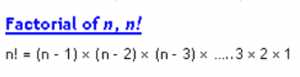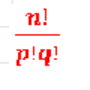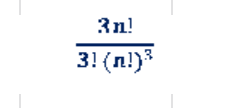## Factorial of n,n! :

The Combinations formula:## Important formulas on Combinations:

nc0 = 1

ncn = 1

ncr = ncn-r

The number of groups (combinations) of n different things taking any number at a time is:

nc0 + nc1 + nc2 +â€¦.. ncn = 2n â€“ 1

• The number of ways (alternatively groups or combinations) in which n things can be divided into p things and q things is• The number of ways (alternatively groups or combinations) in which 3n can be divided equally into three distinct groups each having n things is• The number of ways (alternatively groups or combinations) in which 3n can be divided equally into three identical groups each having n things isInsert math as
$${}$$